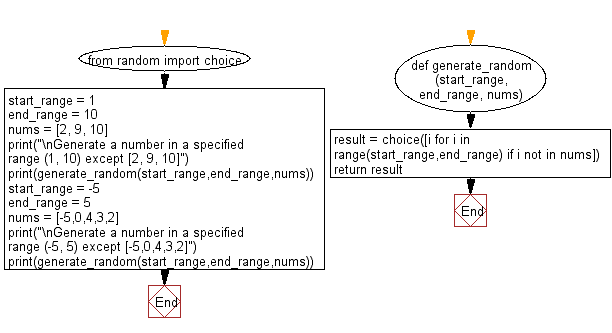﻿ Python: Generate a number in a specified range except some specific numbers - w3resource# Python: Generate a number in a specified range except some specific numbers

## Python List: Exercise - 145 with Solution

Write a Python program to generate a number in a specified range except some specific numbers.

Sample Solution:

Python Code:

``````from random import choice
def generate_random(start_range, end_range, nums):
result = choice([i for i in range(start_range,end_range) if i not in nums])
return result

start_range = 1
end_range = 10
nums = [2, 9, 10]
print("\nGenerate a number in a specified range (1, 10) except [2, 9, 10]")
print(generate_random(start_range,end_range,nums))

start_range = -5
end_range = 5
nums = [-5,0,4,3,2]

print("\nGenerate a number in a specified range (-5, 5) except [-5,0,4,3,2]")
print(generate_random(start_range,end_range,nums))
```
```

Sample Output:

```Generate a number in a specified range (1, 10) except [2, 9, 10]
7

Generate a number in a specified range (-5, 5) except [-5,0,4,3,2]
-4
```

Flowchart:## Visualize Python code execution:

The following tool visualize what the computer is doing step-by-step as it executes the said program:

Python Code Editor:

Have another way to solve this solution? Contribute your code (and comments) through Disqus.

What is the difficulty level of this exercise?

Test your Python skills with w3resource's quiz

﻿

## Python: Tips of the Day

```print(2_000_000)
```2000000